# forecast

Forecast vector autoregression (VAR) model responses

## Syntax

``Y = forecast(Mdl,numperiods,Y0)``
``Y = forecast(Mdl,numperiods,Y0,Name=Value)``
``````[Y,YMSE] = forecast(___)``````
``Tbl2 = forecast(Mdl,numperiods,Tbl1)``
``Tbl2 = forecast(Mdl,numperiods,Tbl1,Name=Value)``
``````[Tbl2,YMSE] = forecast(___)``````
``Tbl2 = forecast(Mdl,numperiods,Tbl1,InSample=InSample,ResponseVariables=ResponseVariables)``
``Tbl2 = forecast(Mdl,numperiods,Tbl1,InSample=InSample,ResponseVariables=ResponseVariables,Name=Value)``
``````[Tbl2,YMSE] = forecast(___)``````

## Description

### Conditional and Unconditional Forecasts for Numeric Arrays

example

````Y = forecast(Mdl,numperiods,Y0)` returns a numeric array containing paths of minimum mean squared error (MMSE) multivariate response forecasts `Y` over a length `numperiods` forecast horizon, using the fully specified VAR(p) model `Mdl`. The forecasted responses represent the continuation of the presample data in the numeric array `Y0`.```

example

````Y = forecast(Mdl,numperiods,Y0,Name=Value)` uses additional options specified by one or more name-value arguments. `forecast` returns numeric arrays when all optional input data are numeric arrays. For example, `forecast(Mdl,10,Y0,X=Exo)` returns a numeric array containing a 10-period forecasted response path from `Mdl` and the numeric matrix of presample response data `Y0`, and specifies the numeric matrix of future predictor data for the model regression component in the forecast horizon `Exo`.To produce a conditional forecast, specify future response data in a numeric array by using the `YF` name-value argument.```

example

``````[Y,YMSE] = forecast(___)``` also returns the corresponding forecast mean squared error (MSE) matrices `YMSE` of each forecasted multivariate response using any input argument combination in the previous syntaxes.```

### Unconditional Forecasts for Tables and Timetables

example

````Tbl2 = forecast(Mdl,numperiods,Tbl1)` returns the table or timetable `Tbl2` containing the length `numperiods` paths of multivariate MMSE response variable forecasts, which result from computing unconditional forecasts from the VAR model `Mdl`. `forecast` uses the table or timetable of presample data `Tbl1` to initialize the response series. (since R2022b)`forecast` selects the variables in `Mdl.SeriesNames` to forecast, or it selects all variables in `Tbl1`. To select different response variables in `Tbl1` to forecast, use the `PresampleResponseVariables` name-value argument.```

example

````Tbl2 = forecast(Mdl,numperiods,Tbl1,Name=Value)` uses additional options specified by one or more name-value arguments. For example, ```forecast(Mdl,10,Tbl1,PresampleResponseVariables=["GDP" "CPI"])``` returns a timetable of response variables containing their unconditional forecasts from the VAR model `Mdl`, initialized by the data in the `GDP` and `CPI` variables of the timetable of presample data in `Tbl1`. (since R2022b)```

example

``````[Tbl2,YMSE] = forecast(___)``` also returns the corresponding forecast MSE matrices `YMSE` of each forecasted multivariate response using any input argument combination in the previous two syntaxes. (since R2022b)```

### Conditional Forecasts for Tables and Timetables

example

````Tbl2 = forecast(Mdl,numperiods,Tbl1,InSample=InSample,ResponseVariables=ResponseVariables)` returns the table or timetable `Tbl2` containing the length `numperiods` paths of multivariate MMSE response variable forecasts and corresponding forecast MSEs, which result from computing conditional forecasts from the VAR model `Mdl`. `forecast` uses the table or timetable of presample data `Tbl1` to initialize the response series. `InSample` is a table or timetable of future data in the forecast horizon that `forecast` uses to compute conditional forecasts and `ResponseVariables` specifies the response variables in `InSample`. (since R2022b)```

example

````Tbl2 = forecast(Mdl,numperiods,Tbl1,InSample=InSample,ResponseVariables=ResponseVariables,Name=Value)` uses additional options specified by one or more name-value arguments. (since R2022b)```

example

``````[Tbl2,YMSE] = forecast(___)``` also returns the corresponding forecast MSE matrices `YMSE` of each forecasted multivariate response using any input argument combination in the previous two syntaxes. (since R2022b)```

## Examples

collapse all

Fit a VAR(4) model to the consumer price index (CPI) and unemployment rate. Then, forecast unconditional MMSE responses from the estimated model. Supply all required data in numeric matrices.

Load the `Data_USEconModel` data set.

```load Data_USEconModel dts = datetime(dates,ConvertFrom="datenum");```

Plot the two series on separate plots.

```figure plot(dts,DataTimeTable.CPIAUCSL); title("Consumer Price Index") ylabel("Index") xlabel("Date")``````figure plot(dts,DataTimeTable.UNRATE); title("Unemployment Rate") ylabel("Percent") xlabel("Date")```Stabilize the CPI by converting it to a series of growth rates. Synchronize the two series by removing the first observation from the unemployment rate series.

```RCPI = price2ret(DataTimeTable.CPIAUCSL); UNRATE = DataTimeTable.UNRATE(2:end); dts = dts(2:end); EstY = [RCPI UNRATE];```

Create a default VAR(4) model by using the shorthand syntax.

`Mdl = varm(2,4);`

Estimate the model using the entire data set.

`EstMdl = estimate(Mdl,EstY);`

`EstMdl` is a fully specified, estimated `varm` model object.

Forecast responses from the estimated model over a three-year horizon. Specify the entire data set as presample observations.

```numperiods = 12; Y0 = EstY; Y = forecast(EstMdl,numperiods,Y0);```

`Y` is a 12-by-2 matrix of forecasted responses. The first and second columns contain the forecasted CPI growth rate and unemployment rate, respectively.

Plot the forecasted responses and the last 50 true responses.

```fh = dateshift(dts(end),"end","quarter",1:numperiods); figure h1 = plot(dts((end-49):end),EstY((end-49):end,1)); hold on h2 = plot(fh,Y(:,1)); title("CPI Growth Rate") ylabel("Growth Rate") xlabel("Date") h = gca; fill([dts(end) fh([end end]) dts(end)],h.YLim([1 1 2 2]),"k", ... FaceAlpha=0.1,EdgeColo="none"); legend([h1 h2],"Data","Forecast") hold off```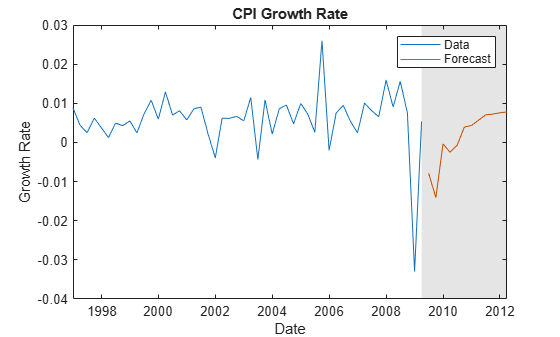```figure h1 = plot(dts((end-49):end),EstY((end-49):end,2)); hold on h2 = plot(fh,Y(:,2)); title("Unemployment Rate") ylabel("Percent") xlabel("Date") h = gca; fill([dts(end) fh([end end]) dts(end)],h.YLim([1 1 2 2]),"k", ... FaceAlpha=0.1,EdgeColo="none"); legend([h1 h2],"True","Forecast",Location="northwest") hold off```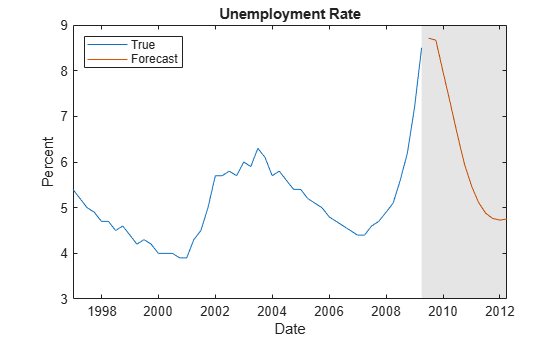This example is based on Return Matrix of VAR Model Forecasts. Forecast MMSE responses of the consumer price index (CPI) growth rate four quarters beyond the sampling data, given that the unemployment rate is 8% for each future quarter in the forecast horizon.

Load the `Data_USEconModel` data set.

```load Data_USEconModel dts = datetime(dates,ConvertFrom="datenum");```

Stabilize the CPI by converting it to a series of growth rates. Synchronize the two series by removing the first observation from the unemployment rate series.

```RCPI = price2ret(DataTimeTable.CPIAUCSL); UNRATE = DataTimeTable.UNRATE(2:end); dts = dts(2:end); EstY = [RCPI UNRATE];```

Create a default VAR(4) model using the shorthand syntax. Estimate the model using the entire data set.

```Mdl = varm(2,4); EstMdl = estimate(Mdl,EstY);```

Forecast the CPI growth rate from the estimated model over a one-year horizon, given that the unemployment rate over the next year is 8% each quarter. Create a 2-by-4 matrix `CondYF` containing the conditions in the forecast horizon, in which the first column (corresponding to `RCPI`) is composed of `NaN` values and the second column (corresponding to `UNRATE`) is completely composed of `8`. Supply the future data to `forecast` and specify the entire data set as presample observations.

```numperiods = 4; Y0 = EstY; CondYF = NaN(numperiods,Mdl.NumSeries); CondYF(:,2) = 8; Y = forecast(EstMdl,numperiods,Y0,YF=CondYF)```
```Y = 4×2 -0.0068 8.0000 -0.0121 8.0000 0.0006 8.0000 -0.0045 8.0000 ```

`Y` is a 4-by-2 matrix of the forecasted CPI growth rate series into the next year, with the unemployment rate fixed at 8%.

Analyze forecast accuracy using forecast intervals over a three-year horizon. This example follows from Return Matrix of VAR Model Forecasts.

Load the `Data_USEconModel` data set and preprocess the data.

```load Data_USEconModel dts = datetime(dates,ConvertFrom="datenum"); RCPI = price2ret(DataTimeTable.CPIAUCSL); UNRATE = DataTimeTable.UNRATE(2:end); D = [RCPI UNRATE];```

Estimate a VAR(4) model of the two response series. Reserve the last three years of data.

```bfh = dts(end) - years(3); estIdx = dts < bfh; Mdl = varm(2,4); EstY = D(estIdx,:); EstMdl = estimate(Mdl,EstY);```

Forecast responses from the estimated model over a three-year horizon. Specify the entire data set as presample observations. Return the MSE of the forecasts.

```numperiods = 12; [Y,YMSE] = forecast(EstMdl,numperiods,EstY);```

`Y` is a 12-by-2 matrix of forecasted responses. `YMSE` is a 12-by-1 cell vector of corresponding MSE matrices.

Extract the main diagonal elements from the matrices in each cell of `YMSE`. Apply the square root of the result to obtain standard errors.

```extractMSE = @(x)diag(x)'; MSE = cellfun(extractMSE,YMSE,UniformOutput=false); SE = sqrt(cell2mat(MSE));```

Estimate approximate 95% forecast intervals for each response series.

```YFI = zeros(numperiods,Mdl.NumSeries,2); YFI(:,:,1) = Y - 2*SE; YFI(:,:,2) = Y + 2*SE;```

Plot the forecasted responses and the last 50 true responses.

```figure h1 = plot(dts((end-49):end),RCPI((end-49):end)); hold on; h2 = plot(dts(~estIdx),Y(:,1)); h3 = plot(dts(~estIdx),YFI(:,1,1),"k--"); plot(dts(~estIdx),YFI(:,1,2),"k--"); title("CPI Growth Rate") ylabel("Growth Rate") xlabel("Date") h = gca; fill([bfh h.XLim([2 2]) bfh],h.YLim([1 1 2 2]),"k", ... FaceAlpha=0.1,EdgeColor="none"); legend([h1 h2 h3],"Data","Forecast","95% forecast interval", ... Location="northwest") hold off```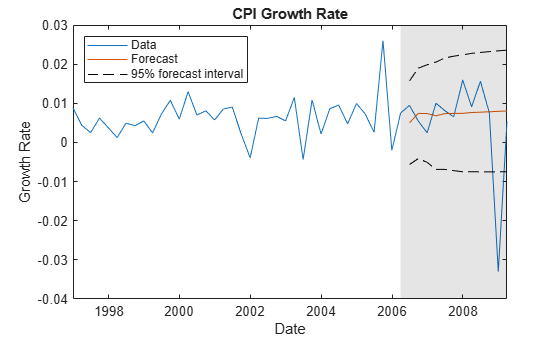```figure h1 = plot(dts((end-49):end),UNRATE((end-49):end)); hold on; h2 = plot(dts(~estIdx),Y(:,2)); h3 = plot(dts(~estIdx),YFI(:,2,1),"k--"); plot(dts(~estIdx),YFI(:,2,2),"k--"); title('Unemployment Rate') ylabel('Percent') xlabel('Date') h = gca; fill([bfh h.XLim([2 2]) bfh],h.YLim([1 1 2 2]),"k", ... FaceAlpha=0.1,EdgeColor="none"); legend([h1 h2 h3],"Data","Forecast","95% forecast interval", ... Location="northwest") hold off```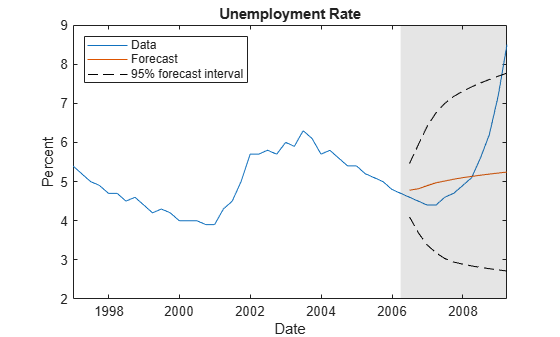Since R2022b

Fit a VAR(4) model to the consumer price index (CPI) and unemployment rate. Then, forecast unconditional MMSE responses from the estimated model. Supply all required data in timetables. This example is based on Return Response Series in Matrix from Unconditional Simulation.

Load the `Data_USEconModel` data set. Compute the CPI growth rate. Because the growth rate calculation consumes the earliest observation, include the rate variable in the timetable by prepending the series with `NaN`.

```load Data_USEconModel DataTimeTable.RCPI = [NaN; price2ret(DataTimeTable.CPIAUCSL)];```

Prepare Timetable for Estimation

When you plan to supply a timetable directly to `estimate`, you must ensure it has all the following characteristics:

• All selected response variables are numeric and do not contain any missing values.

• The timestamps in the `Time` variable are regular, and they are ascending or descending.

Remove all missing values from the table, relative to the CPI rate (`RCPI`) and unemployment rate (`UNRATE`) series.

```varnames = ["RCPI" "UNRATE"]; DTT = rmmissing(DataTimeTable,DataVariables=varnames); numobs = height(DTT)```
```numobs = 245 ```

`rmmissing` removes the four initial missing observations from the `DataTimeTable` to create a sub-table `DTT`. The variables `RCPI` and `UNRATE` of `DTT` do not have any missing observations.

Determine whether the sampling timestamps have a regular frequency and are sorted.

`areTimestampsRegular = isregular(DTT,"quarters")`
```areTimestampsRegular = logical 0 ```
`areTimestampsSorted = issorted(DTT.Time)`
```areTimestampsSorted = logical 1 ```

`areTimestampsRegular = 0` indicates that the timestamps of `DTT` are irregular. `areTimestampsSorted = 1` indicates that the timestamps are sorted. Macroeconomic series in this example are timestamped at the end of the month. This quality induces an irregularly measured series.

Remedy the time irregularity by shifting all dates to the first day of the quarter.

```dt = DTT.Time; dt = dateshift(dt,"start","quarter"); DTT.Time = dt; areTimestampsRegular = isregular(DTT,"quarters")```
```areTimestampsRegular = logical 1 ```

`DTT` is regular with respect to time.

Create Model Template for Estimation

Create a default VAR(4) model by using the shorthand syntax. Specify the response variable names.

```Mdl = varm(2,4); Mdl.SeriesNames = varnames;```

Fit Model to Data

Estimate the model. Pass the entire timetable `DTT`. By default, `estimate` selects the response variables in `Mdl.SeriesNames` to fit to the model. Alternatively, you can use the `ResponseVariables` name-value argument.

`EstMdl = estimate(Mdl,DTT);`

Forecast Responses and Compute Forecast MSEs

Forecast responses from the estimated model over a three-year horizon. Specify the entire data set `DTT` as a presample observations.

```numperiods = 12; [Tbl2,YMSE] = forecast(EstMdl,numperiods,DTT); Tbl2```
```Tbl2=12×2 timetable Time RCPI_Responses UNRATE_Responses _____ ______________ ________________ Q2-09 -0.0078947 8.7104 Q3-09 -0.014099 8.6682 Q4-09 -0.00036087 7.9762 Q1-10 -0.0025178 7.3152 Q2-10 -0.00074203 6.6233 Q3-10 0.0039157 5.9685 Q4-10 0.0043404 5.4787 Q1-11 0.0056518 5.1184 Q2-11 0.0070472 4.8808 Q3-11 0.007241 4.7632 Q4-11 0.0075783 4.728 Q1-12 0.0077906 4.7519 ```
`YMSE`
```YMSE=12×1 cell array {2x2 double} {2x2 double} {2x2 double} {2x2 double} {2x2 double} {2x2 double} {2x2 double} {2x2 double} {2x2 double} {2x2 double} {2x2 double} {2x2 double} ```

`Tbl2` is a 12-by-2 timetable of forecasted CPI growth and unemployment rates. Forecast variable names are appended with `_Responses`, for example, `RCPI_Responses` contains the forecasts of `RCPI`. The timestamps of `Tbl2` follow directly from the timestamps of `DTT`, and they have the same sampling frequency.

`YMSE` is a 12-by-1 cell vector of corresponding 2-by-2 forecast MSE matrices for each period in the forecast horizon. For example, the forecast covariance between each response series in period 6 for the forecast horizon (off diagonal of `YMSE{6}`) is –0.0025.

Since R2022b

Estimate a four-degree vector autoregression model including exogenous predictors (VARX(4)) of the consumer price index (CPI), the unemployment rate, and the gross domestic product (GDP). Include a linear regression component containing the current quarter and the last four quarters of government consumption expenditures and investment (GCE). Forecast a response path from the estimated model.

Load the `Data_USEconModel` data set. Compute the real GDP.

```load Data_USEconModel DataTimeTable.RGDP = DataTimeTable.GDP./DataTimeTable.GDPDEF*100;```

Plot all variables on separate plots.

```figure tiledlayout(2,2) nexttile plot(DataTimeTable.Time,DataTimeTable.CPIAUCSL); ylabel("Index") title("Consumer Price Index") nexttile plot(DataTimeTable.Time,DataTimeTable.UNRATE); ylabel("Percent") title("Unemployment Rate") nexttile plot(DataTimeTable.Time,DataTimeTable.RGDP); ylabel("Output") title("Real Gross Domestic Product") nexttile plot(DataTimeTable.Time,DataTimeTable.GCE); ylabel("Billions of \$") title("Government Expenditures")```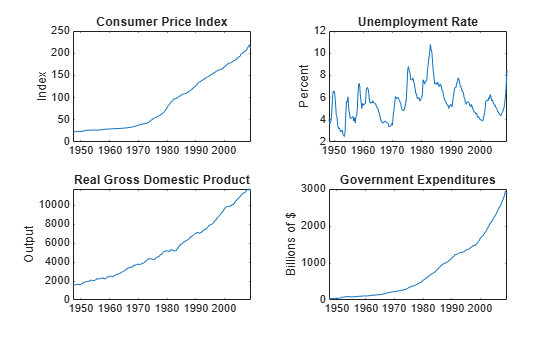Stabilize the CPI, GDP, and GCE by converting each to a series of growth rates. Synchronize the unemployment rate series with the others by removing its first observation.

```varnames = ["CPIAUCSL" "RGDP" "GCE"]; DTT = varfun(@price2ret,DataTimeTable,InputVariables=varnames); DTT.Properties.VariableNames = varnames; DTT.UNRATE = DataTimeTable.UNRATE(2:end);```

Make the time base regular.

```dt = DTT.Time; dt = dateshift(dt,"start","quarter"); DTT.Time = dt;```

Expand the GCE rate series to a matrix that includes the first lagged series through the fourth lag series.

```RGCELags = lagmatrix(DTT,1:4,DataVariables="GCE"); DTT = [DTT RGCELags]; DTT = rmmissing(DTT);```

Create separate presample and estimation sample data sets. The presample contains the earliest `p` = `4` observations, and the estimation sample contains the rest of the data.

```p = 4; PS = DTT(1:p,:); InSample = DTT((p+1):end,:); respnames = ["CPIAUCSL" "UNRATE" "RGDP"]; idx = endsWith(InSample.Properties.VariableNames,"GCE"); prednames = InSample.Properties.VariableNames(idx);```

Create a default VAR(4) model by using the shorthand syntax. Specify the response variable names.

```Mdl = varm(3,p); Mdl.SeriesNames = respnames;```

Estimate the model using all but the last three years of data. Specify the GCE matrix as data for the regression component.

```bfh = DTT.Time(end) - years(3); estIdx = DTT.Time < bfh; EstMdl = estimate(Mdl,DTT(estIdx,:),ResponseVariables=respnames, ... PredictorVariables=prednames);```

Forecast a path of quarterly responses three years into the future.

```numperiods = 12; Tbl1 = DTT(estIdx,:); Tbl2 = forecast(EstMdl,numperiods,Tbl1,InSample=DTT(~estIdx,:), ... PredictorVariables=prednames);```

`Tbl1` is a 12-by-3 timetable of forecasted responses. Variables names correspond to the response variable names in `respnames` appended with `_Responses`.

Plot the forecasted responses and the last 50 true responses.

```figure tiledlayout(2,2) for j = 1:Mdl.NumSeries nexttile h1 = plot(DTT.Time((end-49):end),DTT{(end-49):end,respnames(j)}); hold on h2 = plot(DTT.Time(~estIdx),Tbl2{:,respnames(j)+"_Responses"}); title(respnames(j)) h = gca; fill([bfh h.XLim([2 2]) bfh],h.YLim([1 1 2 2]),"k", ... FaceAlpha=0.1,EdgeColor="none"); hold off end hl = legend([h1 h2],["Data" "Forecast"]); hl.Position = [0.6 0.25 hl.Position(3:4)];```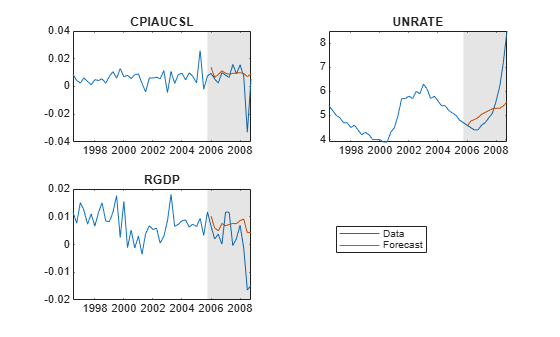Since R2022b

Compute conditional forecasts of the VAR model in Return Timetable of Responses and Innovations from Unconditional Simulation, in which economists hypothesize that the unemployment rate is 6% for 15 quarters after the end of the sample (from Q2 of 2009 through Q4 of 2012).

Load the `Data_USEconModel` data set. Compute the CPI growth rate. Because the growth rate calculation consumes the earliest observation, include the rate variable in the timetable by prepending the series with `NaN`.

```load Data_USEconModel DataTimeTable.RCPI = [NaN; price2ret(DataTimeTable.CPIAUCSL)];```

Prepare Timetable for Estimation

Remove all missing values from the table, relative to the CPI rate (`RCPI`) and unemployment rate (`UNRATE`) series.

```varnames = ["RCPI" "UNRATE"]; DTT = rmmissing(DataTimeTable,DataVariables=varnames);```

Remedy the time irregularity by shifting all dates to the first day of the quarter.

```dt = DTT.Time; dt = dateshift(dt,"start","quarter"); DTT.Time = dt;```

Create Model Template for Estimation

Create a default VAR(4) model using the shorthand syntax. Specify the response variable names.

```p = 4; Mdl = varm(2,p); Mdl.SeriesNames = varnames;```

Fit Model to Data

Estimate the model. Pass the entire timetable `DTT`. By default, `estimate` selects the response variables in `Mdl.SeriesNames` to fit to the model. Alternatively, you can use the `ResponseVariables` name-value argument.

`EstMdl = estimate(Mdl,DTT);`

Prepare for Conditional Forecast of Estimated Model

Suppose economists hypothesize that the unemployment rate will be at 6% for the next 15 quarters.

Create a timetable with the following qualities:

• The timestamps are regular with respect to the estimation sample timestamps and they are ordered from Q2 of 2009 through Q4 of 2012.

• The variable `RCPI` (and, consequently, all other variables in `DTT`) is a 15-by-1 vector of `NaN` values.

• The variable `UNRATE` is a 15-by-1 vector, where each element is 6.

```numperiods = 15; fhdt = DTT.Time(end) + calquarters(1:numperiods); DTTCondF = retime(DTT,fhdt,"fillwithmissing"); DTTCondF.UNRATE = ones(numperiods,1)*6;```

`DTTCondF` is a 15-by-15 timetable that follows directly, in time, from `DTT`, and both timetables have the same variables. All variables in `DTTCondF` contain `NaN` values, except for `UNRATE`, which is a vector composed of the value 6.

Compute Conditional Forecast of Estimated Model

Forecast the CPI growth rate given the hypothesis by supplying the conditioning data `DTTCondF` and specifying the response variable names. Supply the estimation sample as a presample to initialize the model.

```rng(1) % For reproducibility Tbl2 = forecast(EstMdl,numperiods,DTT,InSample=DTTCondF, ... ResponseVariables=EstMdl.SeriesNames); size(Tbl2)```
```ans = 1×2 15 17 ```
```idx = endsWith(Tbl2.Properties.VariableNames,"_Responses"); head(Tbl2(:,idx))```
``` Time RCPI_Responses UNRATE_Responses _____ ______________ ________________ Q2-09 -0.0035362 6 Q3-09 -0.0061302 6 Q4-09 0.0066157 6 Q1-10 -0.0018704 6 Q2-10 3.7558e-05 6 Q3-10 0.003859 6 Q4-10 0.002009 6 Q1-11 0.0033291 6 ```

`Tbl2` is a 15-by-17 timetable of all variables in `DTTCondF` and the `RCPI` forecasts given `UNRATE` is 6% for the next 15 quarters. `RCPI_Responses` contains the forecast path. `UNRATE_Responses` is a vector composed of the value 6. All other variables in `Tbl2` are the variables and their values in `DTTCondF`.

Plot the CPI growth rate forecast with the final few values of the estimation sample data.

```figure h1 = plot(DTT.Time((end-30):end),DTT.RCPI((end-30):end)); hold on h2 = plot(Tbl2.Time,Tbl2.RCPI_Responses); xline(Tbl2.Time(1),"r--",LineWidth=2) hold off title("RCPI Forecast") legend([h1 h2(1)],["Observed" "Forecasted"], ... Location="best")```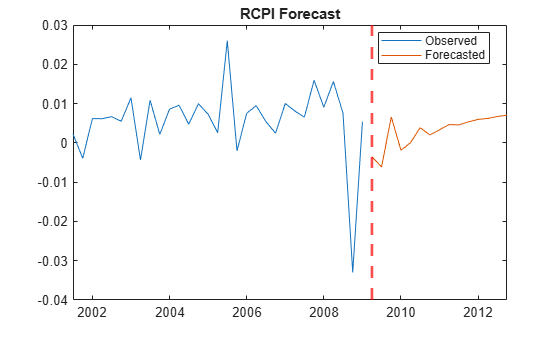Since R2022b

Compute conditional forecasts of the VAR model in Return Timetable of Responses and Innovations from Unconditional Simulation, in which economists make several hypotheses on the value of the unemployment rate at a forecast horizon of one year.

Load the `Data_USEconModel` data set. Preprocess the response variables.

```load Data_USEconModel DataTimeTable.RCPI = [NaN; price2ret(DataTimeTable.CPIAUCSL)];```

Prepare the timetable for estimation.

```varnames = ["RCPI" "UNRATE"]; DTT = rmmissing(DataTimeTable,DataVariables=varnames); dt = DTT.Time; dt = dateshift(dt,"start","quarter"); DTT.Time = dt;```

Estimate the VAR(4) model.

```p = 4; Mdl = varm(2,p); Mdl.SeriesNames = varnames; EstMdl = estimate(Mdl,DTT);```

Consider generating several forecast paths of the CPI growth rate assuming the unemployment rate is 1%, 4%, 5%, 8%, and 10% percent throughout the forecast horizon.

Create a timetable with the following qualities:

• The timestamps are regular with respect to the estimation sample timestamps and they are ordered from Q2 of 2009 through Q1 of 2010.

• The variable `UNRATE` is a 4-by-5 matrix, where each column is composed of each of the assumptions on the value of the unemployment rate in the forecast horizon; the elements of the first column are 1, elements of the second column are 4, and so on.

• The variable `RCPI` is a 4-by-5 matrix of `NaN` values to be filled with forecasted paths.

• All other variables are `NaN`-valued vectors.

```numperiods = 4; fhdt = DTT.Time(end) + calquarters(1:numperiods); DTTCondF = retime(DTT,fhdt,"fillwithmissing"); DTTCondF.UNRATE = ones(numperiods,1)*[1 4 5 8 10]; DTTCondF.RCPI = nan(numperiods,width(DTTCondF.UNRATE));```

`DTTCondF` is a 4-by-15 timetable that follows directly, in time, from `DTT`, and both timetables have the same variables. All variables in `DTTCondF` contain `NaN` values, except for `UNRATE`, which is a 4-by-5 matrix of the hypothesized values of the unemployment rate in the forecast horizon.

Forecast the CPI growth rate given the hypotheses by supplying the conditioning data `DTTCondF` and specifying the response variable names. Supply the estimation sample as a presample to initialize the model. Return the forecast MSE matrices.

```rng(1) % For reproducibility [Tbl2,YMSE] = forecast(EstMdl,numperiods,DTT,InSample=DTTCondF, ... ResponseVariables=EstMdl.SeriesNames); size(Tbl2)```
```ans = 1×2 4 17 ```
```idx = endsWith(Tbl2.Properties.VariableNames,"_Responses"); head(Tbl2(:,idx))```
``` Time RCPI_Responses UNRATE_Responses _____ _____________________________________________________________________ _________________________ Q2-09 0.0045044 -0.00031993 -0.001928 -0.0067524 -0.0099686 1 4 5 8 10 Q3-09 0.0087271 -0.00018729 -0.0031588 -0.012073 -0.018016 1 4 5 8 10 Q4-09 0.021614 0.012615 0.0096155 0.00061625 -0.0053833 1 4 5 8 10 Q1-10 0.0045863 0.00071227 -0.00057906 -0.0044531 -0.0070357 1 4 5 8 10 ```
`YMSE`
```YMSE=4×1 cell array {2x2 double} {2x2 double} {2x2 double} {2x2 double} ```

`Tbl2` is a 4-by-17 timetable of all variables in `DTTCondF`. The `RCPI` forecasts, stored in the variable `RCPI_Responses`, is a 4-by-5 matrix of five forecast paths. Each path uses the corresponding assumption about the value of unemployment rate in `UNRATE_Responses`.

`YMSE` is a 4-by-1 cell vector of forecast MSE matrices for each period in the forecast horizon. The MSE matrices apply to each forecast path, and all elements of each matrix corresponding to the conditioning variable are 0.

## Input Arguments

collapse all

VAR model, specified as a `varm` model object created by `varm` or `estimate`. `Mdl` must be fully specified.

Forecast horizon, or the number of time points in the forecast period, specified as a positive integer.

Data Types: `double`

Presample response data that provides initial values for the forecasts, specified as a `numpreobs`-by-`numseries` numeric matrix or a `numpreobs`-by-`numseries`-by-`numprepaths` numeric array. Use `Y0` only when you supply optional data inputs as numeric arrays.

`numpreobs` is the number of presample observations. `numseries` is the number of response series (`Mdl.NumSeries`). `numprepaths` is the number of presample response paths.

Each row is a presample observation, and measurements in each row, among all pages, occur simultaneously. The last row contains the latest presample observation. `Y0` must have at least `Mdl.P` rows. If you supply more rows than necessary, `forecast` uses the latest `Mdl.P` observations only.

Each column corresponds to the response series name in `Mdl.SeriesNames`.

Pages correspond to separate, independent paths.

• If you compute unconditional forecasts (that is, you do not specify the `YF` name-value argument), `forecast` initializes each forecasted path (page) using the corresponding page of `Y0`. Therefore, the output argument `Y` has `numpaths` = `numprepaths` pages.

• If you compute conditional forecasts by specifying future response data in `YF`: `forecast` takes one of these actions:

• If `Y0` is a matrix, `forecast` initializes each response path (page) in `YF` using the corresponding presample response in `Y0`. Therefore, `numpaths` is the number of paths in `YF`, and all paths in the output argument `Y` derive from common initial conditions.

• If `YF` is a matrix, `forecast` generates `numprepaths` forecast paths, initialized by each presample response path in `Y0`, but the future response data, from which to condition the forecasts, is the same among all paths. Therefore, `numprepaths` is the number of paths in the output argument `Y`, and all paths evolve from possibly different initial conditions.

• Otherwise, `numpaths` is the minimum between `numprepaths` and the number of pages in `YF`, and `forecast` applies `Y0(:,:,j)` to initialize forecasting path `j`, for `j` = 1,…,`numpaths`.

Data Types: `double`

Since R2022b

Presample response data that provides initial values for the forecasts, specified as a table or timetable with `numprevars` variables and `numpreobs` rows. `forecast` returns the forecasted response variable in the output table or timetable `Tbl2`, which is commensurate with `Tbl1`.

Each row is a presample observation, and measurements in each row, among all paths, occur simultaneously. `numpreobs` must be at least `Mdl.P`. If you supply more rows than necessary, `forecast` uses the latest `Mdl.P` observations only.

Each selected response variable is a `numpreobs`-by-`numprepaths` numeric matrix. You can optionally specify `numseries` response variables by using the `PresampleResponseVariables` name-value argument.

Paths (columns) within a particular response variable are independent, but path `j` of all variables correspond, for `j` = 1,…,`numprepaths`. The following conditions apply:

• If you compute unconditional forecasts (that is, you do not specify the `InSample` and `ResponseVariables` name-value arguments), `forecast` initializes each forecasted path per selected response variable using the corresponding path in `Tbl1`. Therefore, each forecasted response variable in the output argument `Tbl2` is a `numperiods`-by-`numprepaths` matrix.

• If you compute conditional forecasts by specifying future response data in `InSample` and corresponding response variables from the data by using `ResponseVariables`, `forecast` takes one of these actions:

• If the selected presample response variables are vectors, `forecast` initializes each forecast path (column) of the selected response variables in `InSample` by using the corresponding presample variable in `Tbl1`. Therefore, all paths in the forecasted response variables evolve from common initial conditions.

• If the selected response variables in `InSample` are vectors, `forecast` generates `numprepaths` forecast paths, initialized by the paths of each selected presample response variable in `Tbl1`, but the future response data, from which to condition the forecasts, is the same among all paths. Therefore, `numpaths` = `numprepaths` is the number of paths in all forecasted response variables, and all paths evolve from possibly different initial conditions.

• Otherwise, `numpaths` is the minimum between `numprepaths` and the number of paths in each selected response variable in `InSample`. For each selected presample and future sample response variable `ResponseK` and each path `j` = 1,…,`numpaths`, `forecast` applies `Tbl1.ResponseK(:,j)` to initialize the conditional forecast for the response data in `Tbl2.ResponseK``(:,j)`.

If `Tbl1` is a timetable, all the following conditions must be true:

• `Tbl1` must represent a sample with a regular datetime time step (see `isregular`).

• The inputs `InSample` and `Tbl1` must be consistent in time such that `Tbl1` immediately precedes `InSample` with respect to the sampling frequency and order.

• The datetime vector of sample timestamps `Tbl1.Time` must be ascending or descending.

If `Tbl1` is a table, the last row contains the latest presample observation.

Since R2022b

Future time series response or predictor data, specified as a table or timetable. `InSample` contains `numvars` variables, including `numseries` response variables yt or `numpreds` predictor variables xt for the model regression component. You can specify `InSample` only when you specify `Tbl1`.

Use `InSample` in the following situations:

• Perform conditional simulation. You must also supply the response variable names to select response data in `InSample` by using the `ResponseVariables` name-value argument.

• Supply future predictor data for either unconditional or conditional simulation. To supply predictor data, you must specify predictor variable names in `InSample` by using the `PredictorVariables` name-value argument. Otherwise, `forecast` ignores the model regression component.

Each row corresponds to an observation in the forecast horizon, the first row is the earliest observation, and measurements in each row, among all paths, occur simultaneously. Specifically, row `j` of variable `VariableK` (`InSample.VariableK(j,:)`) contains observations `j` periods into the future, or the `j`-period-ahead forecasts. `InSample` must have at least `numperiods` rows to cover the forecast horizon. If you supply more rows than necessary, `forecast` uses only the first `numperiods` rows.

Each selected response variable is a numeric matrix. For each selected response variable `K`, columns are separate, independent paths. Specifically, path `j` of response variable `ResponseK` captures the state, or knowledge, of `ResponseK` as it evolves from the presample past (for example, `Tbl1.ResponseK`) into the future. For each selected response variable `ResponseK`:

• If the selected presample response variables in `Tbl1` are vectors, `forecast` initializes each forecast path (column) of the selected response variables in `InSample` by using the corresponding presample variable in `Tbl1`. Therefore, all paths in the forecasted response variables of the output `Tbl2` evolve from common initial conditions.

• If the selected response variables in `InSample` are vectors, `forecast` generates `numprepaths` forecast paths, initialized by the paths of each selected presample response variable in `Tbl1`, but the future response data, from which to condition the forecasts, is the same among all paths. Therefore, `numpaths` = `numprepaths` is the number of paths in all forecasted response variables, and all paths evolve from possibly different initial conditions.

• Otherwise, `numpaths` is the minimum between `numprepaths` and the number of paths in each selected response variable in `InSample`. For each selected presample and future sample response variable `ResponseK` and each path `j` = 1,…,`numpaths`, `forecast` applies `Tbl1.ResponseK(:,j)` to initialize the conditional forecast for the response data in `Tbl2.ResponseK``(:,j)`.

Each predictor variable is a numeric vector. All predictor variables are present in the regression component of each response equation and apply to all response paths.

If `InSample` is a timetable, the following conditions apply:

• `InSample` must represent a sample with a regular datetime time step (see `isregular`).

• The datetime vector `InSample.Time` must be ascending or descending.

• `Tbl1` must immediately precede `InSample`, with respect to the sampling frequency.

If `InSample` is a table, the last row contains the latest observation.

Elements of the response variables of `InSample` can be numeric scalars or missing values (indicated by `NaN` values). `forecast` treats numeric scalars as deterministic future responses that are known in advance, for example, set by policy. `forecast` forecasts responses for corresponding `NaN` values conditional on the known values. Elements of selected predictor variables must be numeric scalars.

By default, `forecast` computes conventional MMSE forecasts and forecast MSEs without a regression component in the model (each selected response variable is a `numperiods`-by-`numprepaths` matrix composed of `NaN` values indicating a complete lack of knowledge of the future state of the responses in the forecast horizon).

For more details, see Algorithms.

Example: Consider forecasting one path from a model composed of two response series, `GDP` and `CPI`, three periods into the future. Suppose that you have prior knowledge about some of the future values of the responses, and you want to forecast the unknown responses conditional on your knowledge. Specify `InSample` as a matrix containing the values that you know, and use `NaN` for values you do not know but want to forecast. For example, ```InSample=array2table([2 NaN; 0.1 NaN; NaN NaN],VariableNames=["GDP" "CPI"])``` specifies that you have no knowledge of the future values of `CPI`, but you know that `GDP` is 2, 0.1, and unknown in periods 1, 2, and 3, respectively, in the forecast horizon.

Since R2022b

Variables to select from `InSample` to treat as response variables yt, specified as one of the following data types:

• String vector or cell vector of character vectors containing `numseries` variable names in `InSample.Properties.VariableNames`

• A length `numseries` vector of unique indices (integers) of variables to select from `InSample.Properties.VariableNames`

• A length `numvars` logical vector, where ```ResponseVariables(j) = true``` selects variable `j` from `InSample.Properties.VariableNames`, and `sum(ResponseVariables)` is `numseries`

The selected variables must be numeric vectors (single path) or matrices (columns represent multiple independent paths) of the same width.

To compute conditional forecasts, you must specify `ResponseVariables` to select the response variables in `InSample` for the conditioning data. `ResponseVariables` applies only when you specify `InSample`.

By default, `forecast` computes conventional MMSE forecasts and forecast MSEs.

Example: `ResponseVariables=["GDP" "CPI"]`

Example: `ResponseVariables=[true false true false]` or `ResponseVariable=[1 3]` selects the first and third table variables as the response variables.

Data Types: `double` | `logical` | `char` | `cell` | `string`

### Name-Value Arguments

Specify optional pairs of arguments as `Name1=Value1,...,NameN=ValueN`, where `Name` is the argument name and `Value` is the corresponding value. Name-value arguments must appear after other arguments, but the order of the pairs does not matter.

Before R2021a, use commas to separate each name and value, and enclose `Name` in quotes.

Example: `forecast(Mdl,10,Y0,X=Exo)` returns a numeric array containing a 10-period forecasted response path from `Mdl` and the numeric matrix of presample response data `Y0`, and specifies the numeric matrix of future predictor data for the model regression component in the forecast horizon `Exo`.

Since R2022b

Variables to select from `Tbl1` to use for presample data, specified as one of the following data types:

• String vector or cell vector of character vectors containing `numseries` variable names in `Tbl1.Properties.VariableNames`

• A length `numseries` vector of unique indices (integers) of variables to select from `Tbl1.Properties.VariableNames`

• A length `numprevars` logical vector, where ```PresampleResponseVariables(j) = true ``` selects variable `j` from `Tbl1.Properties.VariableNames`, and `sum(PresampleResponseVariables)` is `numseries`

The selected variables must be numeric vectors and cannot contain missing values (`NaN`).

`PresampleResponseNames` does not need to contain the same names as in `Mdl.SeriesNames`; `forecast` uses the data in selected variable `PresampleResponseVariables(j)` as a presample for `Mdl.SeriesNames(j)`.

If the number of variables in `Tbl1` matches `Mdl.NumSeries`, the default specifies all variables in `Tbl1`. If the number of variables in `Tbl1` exceeds `Mdl.NumSeries`, the default matches variables in `Tbl1` to names in `Mdl.SeriesNames`.

Example: `PresampleResponseVariables=["GDP" "CPI"]`

Example: `PresampleResponseVariables=[true false true false]` or `PresampleResponseVariable=[1 3]` selects the first and third table variables for presample data.

Data Types: `double` | `logical` | `char` | `cell` | `string`

Forecasted time series of predictor data xt to include in the model regression component, specified as a numeric matrix containing `numpreds` columns. Use `X` only when you supply `Y0`.

`numpreds` is the number of predictor variables (`size(Mdl.Beta,2)`).

Each row corresponds to an observation in the forecast horizon, and measurements in each row occur simultaneously. Specifically, row `j` (`X(j,:)`) contains the predictor observations `j` periods into the future, or the `j`-period-ahead forecasts. `X` must have at least `numperiods` rows. If you supply more rows than necessary, `forecast` uses only the earliest `numperiods` observations. The first row contains the earliest observation. `forecast` does not use the regression component in the presample period.

Each column is an individual predictor variable. All predictor variables are present in the regression component of each response equation.

`forecast` applies `X` to each path (page); that is, `X` represents one path of observed predictors.

To maintain model consistency into the forecast horizon, specify forecasted predictors when `Mdl` has a regression component.

By default, `forecast` excludes the regression component, regardless of its presence in `Mdl`.

Data Types: `double`

Future multivariate response series data for conditional forecasting, specified as a numeric matrix or array containing `numseries` columns. Use `YF` only when you supply `Y0`.

Each row corresponds to observations in the forecast horizon, and the first row is the earliest observation. Specifically, row `j` in sample path `k` (`YF(j,:,k)`) contains the responses `j` periods into the future, or the `j`-period-ahead forecasts. `YF` must have at least `numperiods` rows to cover the forecast horizon. If you supply more rows than necessary, `forecast` uses only the first `numperiods` rows.

Each column corresponds to the response variable name in `Mdl.SeriesNames`.

Each page corresponds to a separate sample path. Specifically, path `k` (`YF(:,:,k)`) captures the state, or knowledge, of the response series as they evolve from the presample past (`Y0`) into the future.

• If `YF` is a matrix, `forecast` generates `numprepaths` forecast paths, initialized by each presample response path in `Y0`, but the future response data, from which to condition the forecasts, is the same among all paths. Therefore, `numprepaths` is the number of paths in the output argument `Y`, and all paths evolve from possibly different initial conditions.

• If `Y0` is a matrix, `forecast` initializes each response path (page) in `YF` using the corresponding presample response in `Y0`. Therefore, `numpaths` is the number of paths in `YF`, and all paths in the output argument `Y` derive from common initial conditions.

• Otherwise, `numpaths` is the minimum between `numprepaths` and the number of pages in `YF`, and `forecast` applies `Y0(:,:,j)` to initialize forecasting path `j`, for `j` = 1,…,`numpaths`.

Elements of `YF` can be numeric scalars or missing values (indicated by `NaN` values). `forecast` treats numeric scalars as deterministic future responses that are known in advance, for example, set by policy. `forecast` forecasts responses for corresponding `NaN` values conditional on the known values.

By default, `YF` is an array composed of `NaN` values indicating a complete lack of knowledge of all responses in the forecast horizon. In this case, `forecast` estimates conventional MMSE forecasts.

For more details, see Algorithms.

Example: Consider forecasting one path from a model composed of four response series three periods into the future. Suppose that you have prior knowledge about some of the future values of the responses, and you want to forecast the unknown responses conditional on your knowledge. Specify `YF` as a matrix containing the values that you know, and use `NaN` for values you do not know but want to forecast. For example, ```YF=[NaN 2 5 NaN; NaN NaN 0.1 NaN; NaN NaN NaN NaN]``` specifies that you have no knowledge of the future values of the first and fourth response series; you know the value for period 1 in the second response series, but no other value; and you know the values for periods 1 and 2 in the third response series, but not the value for period 3.

Data Types: `double`

Since R2022b

Variables to select from `InSample` to treat as exogenous predictor variables xt, specified as one of the following data types:

• String vector or cell vector of character vectors containing `numpreds` variable names in `InSample.Properties.VariableNames`

• A length `numpreds` vector of unique indices (integers) of variables to select from `InSample.Properties.VariableNames`

• A length `numvars` logical vector, where `PredictorVariables(j) = true ` selects variable `j` from `InSample.Properties.VariableNames`, and `sum(PredictorVariables)` is `numpreds`

Regardless, selected predictor variable `j` corresponds to the coefficients `Mdl.Beta(:,j)`.

`PredictorVariables` applies only when you specify `InSample`.

The selected variables must be numeric vectors and cannot contain missing values (`NaN`).

By default, `forecast` excludes the regression component, regardless of its presence in `Mdl`.

Example: `PredictorVariables=["M1SL" "TB3MS" "UNRATE"]`

Example: `PredictorVariables=[true false true false]` or `PredictorVariable=[1 3]` selects the first and third table variables as the response variables.

Data Types: `double` | `logical` | `char` | `cell` | `string`

Note

• `NaN` values in `Y0` and `X` indicate missing values. `forecast` removes missing values from the data by list-wise deletion. If `Y0` is a 3-D array, then `forecast` performs these steps:

1. Horizontally concatenate pages to form a `numpreobs`-by-`numpaths*numseries` matrix.

2. Remove any row that contains at least one `NaN` from the concatenated data.

In the case of missing observations, the results obtained from multiple paths of `Y0` can differ from the results obtained from each path individually.

For missing values in `X`, `forecast` removes the corresponding row from each page of `YF`. After row removal from `X` and `YF`, if the number of rows is less than `numperiods`, `forecast` issues an error.

• `forecast` issues an error when selected response variables from `Tbl1` and selected predictor variables from `InSample` contain any missing values.

## Output Arguments

collapse all

MMSE forecasts of the multivariate response series, returned as a `numobs`-by-`numseries` numeric matrix or a `numobs`-by-`numseries`-by-`numpaths` numeric array. `forecast` returns `Y` only when you supply presample data `Y0` as a numeric matrix or array.

`Y` represents the continuation of the presample responses in `Y0`.

Each row is a time point in the simulation horizon. Specifically, row `j` contains the `j`-period-ahead forecasts. Values in a row, among all pages, occur simultaneously. The last row contains the latest forecasted values.

Each column corresponds to the response series name in `Mdl.SeriesNames`.

Pages correspond to separate, independently forecasted paths.

If you specify future responses for conditional forecasting using the `YF` name-value argument, the known values in `YF` appear in the same positions in `Y`. However, `Y` contains forecasted values for the missing observations in `YF`.

Since R2022b

MMSE forecasts of multivariate response series and other variables, returned as a table or timetable, the same data type as `Tbl1`. `forecast` returns `Tbl2` only when you supply the inputs `Tbl1`.

`Tbl2` contains the following variables:

• The forecasted response paths within the `numperiods` length forecast horizon of the selected response series yt. Each forecasted response variable in `Tbl2` is a `numperiods`-by-`numpaths` numeric matrix, where `numpaths` depends on the number of response paths in the specified presample or future sample data (see `Tbl1` or `InSample`). Each row corresponds to a time in the forecast horizon and each column corresponds to a separate path. `forecast` names the forecasted response variable `ResponseK` `ResponseK_Responses`. For example, if `Mdl.Series(K)` is `GDP`, `Tbl2` contains a variable for the corresponding forecasted response with the name `GDP_Responses`. If you specify `ResponseVariables`, `ResponseK` is `ResponseVariable(K)`. Otherwise, `ResponseK` is `PresampleResponseVariable(K)`.

• If you specify `InSample`, all specified future response variables.

If `Tbl2` is a timetable, the following conditions hold:

• The row order of `Tbl2`, either ascending or descending, matches the row order of `InSample`, when you specify it. If you do not specify `InSample`, the row order of `Tbl2` is the same as the row order `Tbl1`.

• If you specify `InSample`, row times `Tbl2.Time` are `InSample.Time(1:numperiods)`. Otherwise, `Tbl2.Time(1)` is the next time after `Tbl1(end)` relative to the sampling frequency, and `Tbl2.Time(2:numperiods)` are the following times relative to the sampling frequency.

MSE matrices of the forecasted responses, returned as a `numperiods`-by-1 cell vector of `numseries`-by-`numseries` numeric matrices. Cells of `YMSE` compose a time series of forecast error covariance matrices. Cell `j` contains the `j`-period-ahead MSE matrix.

`YMSE` is identical for all paths.

Because `forecast` treats predictor variables in `X` as exogenous and nonstochastic, `YMSE` reflects the error covariance associated with the autoregressive component of the input model `Mdl` only.

## Algorithms

• `forecast` estimates unconditional forecasts using the equation

`${\stackrel{^}{y}}_{t}={\stackrel{^}{\Phi }}_{1}{\stackrel{^}{y}}_{t-1}+...+{\stackrel{^}{\Phi }}_{p}{\stackrel{^}{y}}_{t-p}+\stackrel{^}{c}+\stackrel{^}{\delta }t+\stackrel{^}{\beta }{x}_{t},$`

where t = 1,...,`numperiods`. `forecast` filters a `numperiods`-by-`numseries` matrix of zero-valued innovations through `Mdl`. `forecast` uses specified presample innovations (`Y0` or `Tbl1`) wherever necessary.

• `forecast` estimates conditional forecasts using the Kalman filter.

1. `forecast` represents the VAR model `Mdl` as a state-space model (`ssm` model object) without observation error.

2. `forecast` filters the forecast data `YF` through the state-space model. At period t in the forecast horizon, any unknown response is

`${\stackrel{^}{y}}_{t}={\stackrel{^}{\Phi }}_{1}{\stackrel{^}{y}}_{t-1}+...+{\stackrel{^}{\Phi }}_{p}{\stackrel{^}{y}}_{t-p}+\stackrel{^}{c}+\stackrel{^}{\delta }t+\stackrel{^}{\beta }{x}_{t},$`

where ${\stackrel{^}{y}}_{s},$ s < t, is the filtered estimate of y from period s in the forecast horizon. `forecast` uses specified presample values in `Y0` or `Tbl1` for periods before the forecast horizon.

For more details, see `filter` and , pp. 612 and 615.

• The way `forecast` determines `numpaths`, the number of paths (pages) in the output argument `Y`, or the number of paths (columns) in the forecasted response variables in the output argument `Tbl2`, depends on the forecast type.

• If you estimate unconditional forecasts, which means you do not specify the `YF` name-value argument, or `InSample` and `ResponseVariables` name-value arguments, `numpaths` is the number of paths in the `Y0` or `Tbl1` input argument.

• If you estimate conditional forecasts and the presample data `Y0` and future sample data `YF`, or response variables in `Tbl1` and `InSample` have more than one path, `numpaths` is the fewest number of paths between the presample and future sample response data. Consequently, `forecast` uses only the first `numpaths` paths of each response variable for each input.

• If you estimate conditional forecasts and either `Y0` or `YF`, or response variables in `Tbl1` or `InSample` have one path, `numpaths` is the number of pages in the array with the most pages. `forecast` uses the variables with one path to produce each output path.

• `forecast` sets the time origin of models that include linear time trends t0 to `numpreobs``Mdl.P` (after removing missing values), where `numpreobs` is the number of presample observations. Therefore, the times in the trend component are t = t0 + 1, t0 + 2,..., t0 + `numpreobs`. This convention is consistent with the default behavior of model estimation in which `estimate` removes the first `Mdl.P` responses, reducing the effective sample size. Although `forecast` explicitly uses the first `Mdl.P` presample responses in `Y0` or `Tbl1` to initialize the model, the total number of usable observations determines t0. An observation in `Y0` is usable if it does not contain a `NaN`.

 Hamilton, James D. Time Series Analysis. Princeton, NJ: Princeton University Press, 1994.

 Johansen, S. Likelihood-Based Inference in Cointegrated Vector Autoregressive Models. Oxford: Oxford University Press, 1995.

 Juselius, K. The Cointegrated VAR Model. Oxford: Oxford University Press, 2006.

 Lütkepohl, H. New Introduction to Multiple Time Series Analysis. Berlin: Springer, 2005.